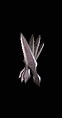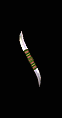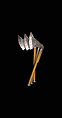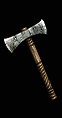﻿ Throwing Weapons (Diablo2) - 【暗黑百科】DiabloWiki_凯恩之角

# Throwing Weapons (Diablo2)

## 普通

 飞刀Throwing Knife投掷武器套装: -暗金: -投掷伤害=4-9 (6.5)最大/最小伤害=2-3 （2.5）攻击距离=1基础武器速度=0需要力量= -需要敏捷= 21最大数量=160最大可打孔数= -品质等级需要等级=2
 平衡小刀Balanced Knife投掷武器套装: -暗金: -投掷伤害=6-11(8.5)最大/最小伤害=1-8 （4.5）攻击距离=1基础武器速度=-20需要力量= -需要敏捷= 51最大数量=160最大可打孔数= -品质等级需要等级=13
 飞斧Throwing Axe投掷武器套装: -暗金: -投掷伤害=8-12 (10)最大/最小伤害=4-7 （5.5）攻击距离=1基础武器速度=10需要力量= -需要敏捷= 40最大数量=130最大可打孔数= -品质等级需要等级=7
 平衡斧Balanced Axe投掷武器套装: -暗金: -投掷伤害=12-15 (13.5)最大/最小伤害=5-10 （7.5）攻击距离=1基础武器速度=-10需要力量= -需要敏捷= 57最大数量=130最大可打孔数= -品质等级需要等级=16

## 扩展

 战斗飞镖Battle Dart投掷武器套装: -暗金: 死亡碎片投掷伤害=11-24 (17.5)最大/最小伤害=8-16 （12）攻击距离=1基础武器速度=0需要力量= 25需要敏捷= 52最大数量=160最大可打孔数= -品质等级需要等级=31/19
 巨战飞镖War Dart投掷武器套装: -暗金: -投掷伤害=14-27(20.5)最大/最小伤害=6-24 （15）攻击距离=1基础武器速度=-20需要力量=25需要敏捷= 97最大数量=160最大可打孔数= -品质等级需要等级=39/25
 法兰飞斧Francisca投掷武器套装: -暗金: 圆凿投掷伤害=18-33 (25.5)最大/最小伤害=11-22（16.5）攻击距离=1基础武器速度=10需要力量= 25需要敏捷= 80最大数量=130最大可打孔数= -品质等级需要等级=34/22
 短战戟Hurbat投掷武器套装: -暗金: -投掷伤害=24-34 (39)最大/最小伤害=5-10 （7.5）攻击距离=1基础武器速度=-10需要力量=25需要敏捷=106最大数量=130最大可打孔数= -品质等级需要等级=41/25

## 精华

 飞刀Flying Knife投掷武器套装: -暗金: -投掷伤害=23-54(38.5)最大/最小伤害=23-54 (38.5) （12）攻击距离=1基础武器速度=0需要力量= 48需要敏捷=141最大数量=200最大可打孔数= -品质等级需要等级=64/48
 翼刀Winged Knife投掷武器套装: -暗金: 战争之鸟投掷伤害=23-39(31)最大/最小伤害=27-35 （31）攻击距离=1基础武器速度=-20需要力量=45需要敏捷= 142最大数量=160最大可打孔数= -品质等级需要等级=77/57
 飞斧Flying Axe投掷武器套装: -暗金: 碎片贪噬者投掷伤害=15-66 (40.5)最大/最小伤害=17-65（41）攻击距离=1基础武器速度=10需要力量=88需要敏捷= 108最大数量=180最大可打孔数= -品质等级需要等级=56/42
 翼斧Winged Axe投掷武器套装: -暗金: 撕裂者投掷伤害=7-60 (35.5)最大/最小伤害=11-56 （33.5）攻击距离=1基础武器速度=-10需要力量=96需要敏捷=122最大数量=180最大可打孔数= -品质等级需要等级=96/60